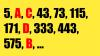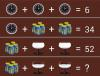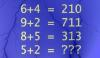BRAIN TEASERSBrain Teasers User Profile

# BrainTeasers Mozgalice

rank
235
points
17
See full ranking list
short ranking list
 233 Art Dexter Antipado 18 234 balakistaiah abbu 18 235 BrainTeasers Mozgalice 17 236 Ilan Amity 17 237 Khoi Nguyen 17
 Calculate A*B-C-D Look at the series (5, A, C, 43, 73, 115, 171, D, 333, 443, 575, B, ...), determine the pattern, and find the unknown values (A, B, C and D) and calculate A*B-C-D!Can you replace the question mark with a number? MATH PUZZLE: Can you replace the question mark with a number?Mathematical Puzzle: IF 6+4=... Mathematical Puzzle: IF 6+4=210, 9+2=711 and 8+5=313 THEN 5+2=?